# Get Employee Information Using VLOOKUP in Excel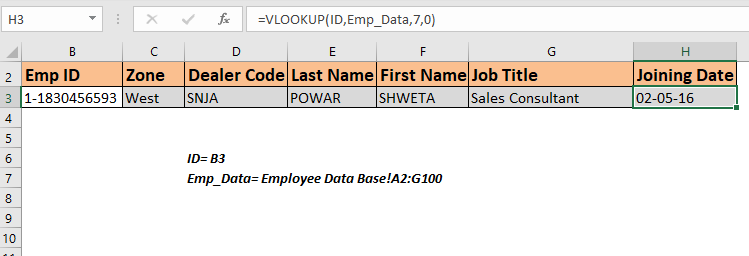To retrieve information from an employee database we can use a VLOOKUP formula. This formula will simply find the unique id and return the information related to that employee ID. If you are new to VLOOKUP functions, this is one of the best VLOOKUP exercises.

So to fetch employee records from the database, we can use this simple VLOOKUP formula:

 =VLOOKUP(id,database,col,0)

ID: it is the unique ID of the employee in the database. We will use it to look up employee information.

Database: It is a table that contains the information of employees. The first column must be the Id.

Col: It is the column number from which you want to retrieve the value.

0 or False: It is used for the exact match VLOOKUP.

Now that we know the generic formula, let's use it as an Example.

## Retrieve Employee Information Using VLOOKUP from Employee Table

We have a table that contains the details of all the employees in an organization on a separate sheet. The first column contains the ID of these employees. I have named this table as emp_data.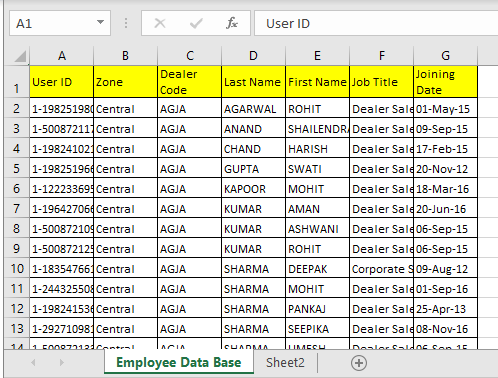Now my search sheet needs to fetch the employee's information whose ID is written in cell B3. I have named B3 as my ID.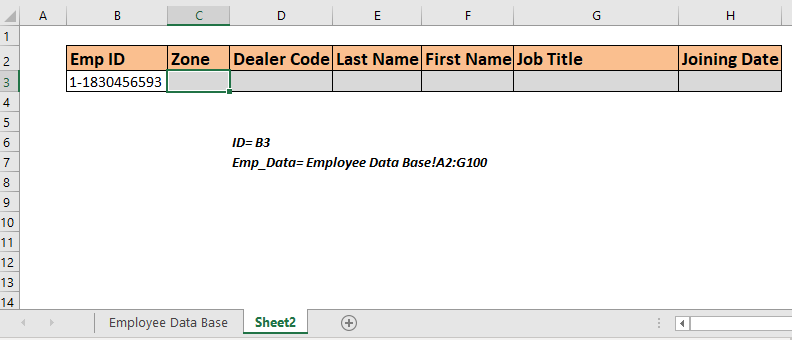For ease of understanding, all the column headings are in exactly the same order as the emp_data  table.

Now write the below formula in cell C3 to retrieve the zone of the employee ID written in B3.

 =VLOOKUP(ID,Emp_Data,2,0)

This will return to the zone of the employee Id 1-1830456593 because column number 2 in the database contains the zone of employees.

Copy this formula in the rest of the cells and change the column number in the formula to get all the information of employees.You can see that all the information related to the mentioned ID in Cell B3. Whichever id you write in Cell B3, all the information will be fetched without making any changes in the formula.

How does this work?

There's nothing tricky. We are simply using the VLOOKUP function to lookup ID and then fetch the mentioned column. Practice VLOOKUP using such data to understand the VLOOKUP more.

## Retrieve Employee Data Using Headings

In the above example, we had all the columns organized in the same order but there will be times when you'll have a database that will have hundreds of columns. In such cases, this method of retrieving employee information won't be good. It would be better if the formula can look at the column heading and fetch data from that column from the employee table.

So to retrieve value from the table using column headings we will use a 2-way lookup method or say dynamic column VLOOKUP.

Use this formula in cell C3 and copy in the rest cells. You don't need to change anything in the formula, everything will be fetched from the emp_data.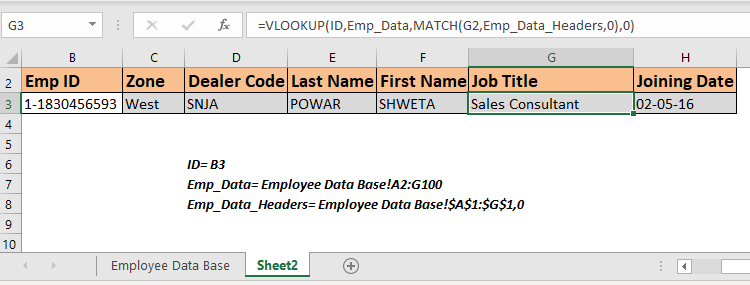This formula simply retrieves all  the information from matched columns. You can jumble the headers in the report, this won't make any difference. Whichever heading is written in the cell above, the respective data contains.

How does this work?

This is simply a dynamic VLOOKUP. You can read about it here. If I explain it here, it will become too large article.

## Retrieve Employee ID with Partial Match

It may happen that you don't remember the whole ID of an employee, but you still want to retrieve the information of some ID. In such cases partial match VLOOKUP is the best solution.

For example, If I know that some id contains 2345 but I don't know the whole Id. If I enter this number in Cell C3, the output will be like.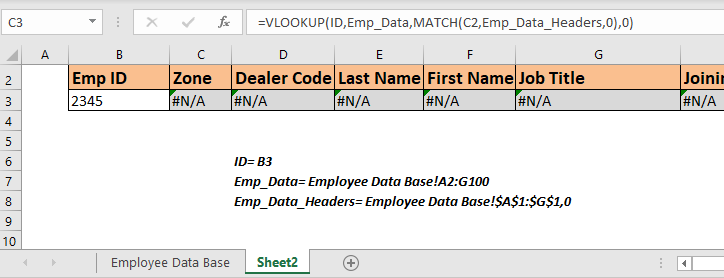We get nothing. Since nothing matches 2345 in the table. Modify the above formula like this.

Copy this in the entire row. Now you have the information of the first employee that contains this number.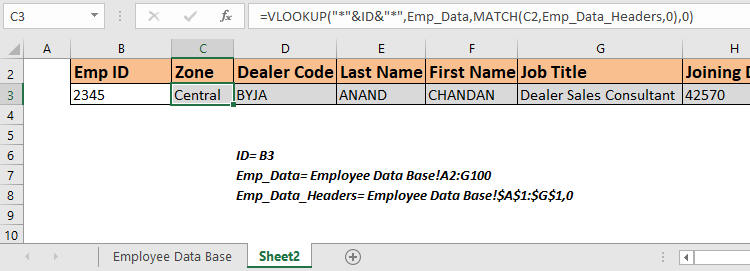Please note that we will get the first ID that contains the matching number in Emp Id column. If any other ID contains the same number, this formula will not retrieve that employee's information.

If you want to get all the employee ids that contain the same number, use the formula that lookups all the matched values.

So yeah guys, this is how you can retrieve employee information from a data table in Excel using VLOOKUP formula. Practice VLOOKUP with such data more and more to understand the VLOOKUP's working.

I hope it was explanatory enough and served your purpose of being here. If you have any doubt regarding this article or any other Excel VBA related doubt, ask me in the comment section below. Till then keep practicing and keep Excelling.

Related Articles

How to Retrieve Latest Price in Excel : It is common to update prices in any business and using the latest prices for any purchase or sales is must. To retrieve the latest price from a list in Excel we use the LOOKUP function. The LOOKUP function fetches the latest price.

VLOOKUP function to calculate grade in Excel : To calculate grades IF and IFS are not the only functions that you can use. The VLOOKUP is more efficient and dynamic for such conditional calculations.To calculate grades using VLOOKUP we can use this formula.

17 Things About Excel VLOOKUP : VLOOKUP is most commonly used for retrieving matched values but VLOOKUP can do a lot more than this. Here are 17 things about VLOOKUP that you should know to use effectively.

LOOKUP the First Text from a List in Excel : The VLOOKUP function works fine with wildcard characters. We can use this to extract the first text value from a given list in excel. Here is the generic formula.

LOOKUP date with last value in list : To retrieve the date that contains the last value we use the LOOKUP function. This function checks for the cell that contains the last value in a vector and then uses that reference to return the date.

Popular Articles:

50 Excel Shortcut to Increase Your Productivity : Get faster at your task. These 50 shortcuts will make you work even faster on Excel.

How to use the COUNTIF function in Excel : Count values with conditions using this amazing function. You don't need to filter your data to count specific values. Countif function is essential to prepare your dashboard.

How to Use SUMIF Function in Excel : This is another dashboard essential function. This helps you sum up values on specific conditions.

How to use the VLOOKUP Function in Excel : This is one of the most used and popular functions of excel that is used to lookup value from different ranges and sheets.

Terms and Conditions of use

The applications/code on this site are distributed as is and without warranties or liability. In no event shall the owner of the copyrights, or the authors of the applications/code be liable for any loss of profit, any problems or any damage resulting from the use or evaluation of the applications/code.